Courses

# Complex Number MCQ Level - 2

## 10 Questions MCQ Test Topic wise Tests for IIT JAM Physics | Complex Number MCQ Level - 2

Description
This mock test of Complex Number MCQ Level - 2 for IIT JAM helps you for every IIT JAM entrance exam. This contains 10 Multiple Choice Questions for IIT JAM Complex Number MCQ Level - 2 (mcq) to study with solutions a complete question bank. The solved questions answers in this Complex Number MCQ Level - 2 quiz give you a good mix of easy questions and tough questions. IIT JAM students definitely take this Complex Number MCQ Level - 2 exercise for a better result in the exam. You can find other Complex Number MCQ Level - 2 extra questions, long questions & short questions for IIT JAM on EduRev as well by searching above.
QUESTION: 1

### The point of intersection the curves arg ( z - i + 2 ) =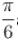and arg ( z + 4 - 3i ) =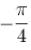is given by

Solution: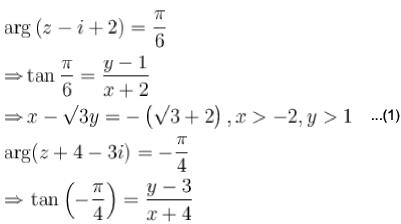⇒ y + x = - 1 ,x > - 4 , y < 3 ...(2)
so, there is no point of intersection.
The correct answer is: None of these

QUESTION: 2

### If 1, α1,α2,α3.........and α8 re nine, ninth roots of unity (taken in counter- clockwise sequence) then | ( 2 - α1 ) (2 - α3) ( 2 - α5 ) ( 2 - α7 ) | is equal to :

Solution:

( x - 1 ) ( x - α1) ( x - α2) ...( x - α8) ≡ x9 - 1
∴ ( 2 - α1 )  ( 2 - α2 )... ( 2 - α8 ) = 2- 1
Now since  2 - αand 2 - α) are conjugates of each other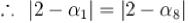similarly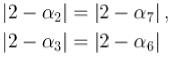and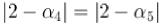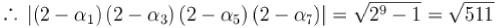The correct answer is: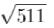QUESTION: 3

### If 'z' is complex number then the locus of ‘z’ satisfying the condition |2z - 1| = | z - 1 | is

Solution: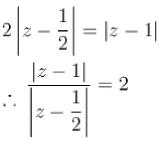or

Let us write z as x+iy
We then have ∣2z−1∣=∣z−1∣
⇒4x2−8x+1+4y2=x2−2x+1+y2
⇒3x2+3y2−6x=0 which is a circle.
So, locus of z is a circle.

QUESTION: 4

If t and c are two complex numbers such that | t |  = 1 and z =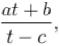z = x + iy , Locus of z is (where a, b are complex numbers)

Solution: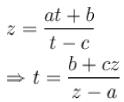|t| = 1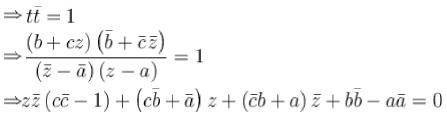QUESTION: 5

If ω is an imaginary cube root of unity, then (1+ ω - ω2 )7 is equal to

Solution: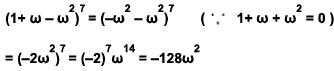T he correct answer is: -128 ω2

QUESTION: 6

The principal argument of the complex number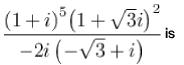Solution: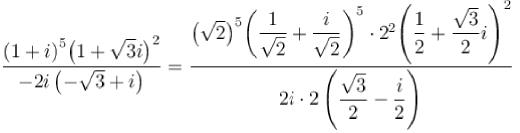∴ argument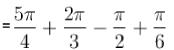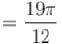∴ principal argument is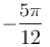The correct answer is: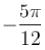QUESTION: 7

If a complex number z satisfies | 2z + 10 + 10i | < 5 √3 - 5, then the least principal argument of z is.

Solution:

Point B has least principal argument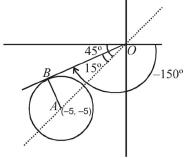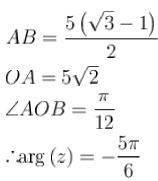The correct answer is: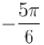QUESTION: 8

Image of the point, whose affix is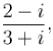in the line (1 + i ) z + ( 1 - i )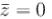is the point whose affix is :

Solution:

Equation of the line is 2x - 2y = 0
i.e. y = x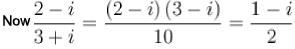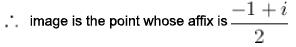The correct answer is :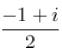QUESTION: 9

Let S denote the set of all complex numbers z satisfying the inequality |z - 5i| < 3.The complex numbers z in S having least positive argument is :

Solution:

See the figure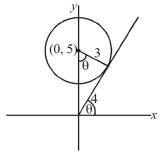|z - 5i| < 3
the point is ( 4 cosθ, 4 sinθ)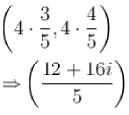The correct answer is: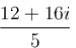QUESTION: 10

Number of solution of the equation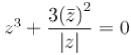where z is a complex number is :

Solution: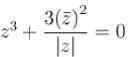Let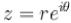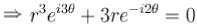Since ‘r ’ cannot be zero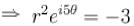which will hold for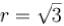and 5 distinct value o f 'O'
Thus there are five solution.